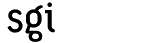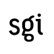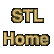1. Introduction to the STL
2. How to use the documentation
3. Containers
1. Concepts
1. General concepts
2. Sequences
3. Associative Containers
2. Container classes
1. Sequences
2. Associative Containers
3. String package
4. rope
6. bitset
4. Iterators
1. Introduction
2. Concepts
3. Iterator Tags
1. Introduction
2. iterator_traits
3. iterator_category
4. distance_type
5. value_type
6. Iterator tag classes
7. Iterator base classes
4. Iterator functions
5. Iterator classes
5. Algorithms
1. Non-mutating algorithms
2. Mutating algorithms
1. copy
2. copy_n
3. copy_backward
4. Swap
5. transform
6. Replace
7. fill
8. fill_n
9. generate
10. generate_n
11. Remove
12. unique
13. unique_copy
14. reverse
15. reverse_copy
16. rotate
17. rotate_copy
18. random_shuffle
19. random_sample
20. random_sample_n
21. partition
22. stable_partition
3. Sorting
1. Sort
2. nth_element
3. Binary search
4. merge
5. inplace_merge
6. Set operations on sorted ranges
7. Heap operations
8. Minimum and maximum
9. lexicographical_compare
10. lexicographical_compare_3way
11. next_permutation
12. prev_permutation
4. Generalized numeric algorithms
6. Function Objects
1. Introduction
2. Concepts
3. Predefined function objects
1. Arithmetic operations
1. plus
2. minus
3. multiplies (formerly called "times")
4. divides
5. modulus
6. negate
2. Comparisons
3. Logical operations
4. Generalized identity operations
5. subtractive_rng
1. binder1st
2. binder2nd
3. ptr_fun
4. pointer_to_unary_function
5. pointer_to_binary_function
6. unary_negate
7. binary_negate
8. unary_compose
9. binary_compose